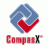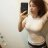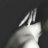# Equity Multiplier (em)

• CompasX#### CompasX

##### Guest
EQUITY MULTIPLIER (EM) shows the amount of assets owned by the firm for each equivalent monetary unit owner claims held by stockholders, i.e., the equity multiplier measures how many dollars of assets an institution supports with each dollar of capital. If a firm is totally financed by equity, the equity multiplier will equal 1.00, while the larger the number the more highly leveraged is the firm. EM compares assets with equity: large values indicate a large amount of debt financing relative to equity. EM, thus, measures financial leverage and represents both profit and risk measurement. EM affects a firm’s profit because it has a multiplier impact on Return on Assets (ROA) to determine the firm’s Return on Equity (ROE). EM is also a risk measure because it reflects how many assets can go into default before a company becomes insolvent. The EM ratio is best compared to industry averages.

T
Trả lời
0
Lượt xem
28
Quảng cáo, rao vặt
Thanh Tuyền cvl
TTrả lời
0
Lượt xem
1KTrả lời
5
Lượt xem
2KTrả lời
0
Lượt xem
3KC
Trả lời
0
Lượt xem
124K
C

Chia sẻ